# Gauss 's Law

## (C) Electric field due to charged solid sphere

• We'll now apply Gauss's law to find the field outside uniformly charged solid sphere of radius R and total charge q.
• In this case Gaussian surface would be a sphere of radius r>R concentric with the charged solid sphere shown below in the figure.From Gauss's law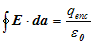where q is the charge enclosed.
• Charge is distributed uniformly over the surface of the sphere. Symmetry allows us to extract E out of the integral sign as magnitude of electric field intensity is same for all points at distance r>R.
• Since electric field points radially outwards we have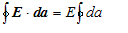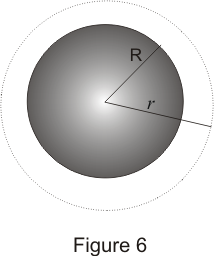also as discussed magnitude of E is constant over Gaussian surface so,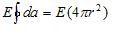where 4πr2 is the surface area of the sphere.
Again from Gauss's law we have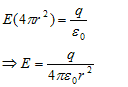THus we see that magnitude of field outside the sphere is exactly the same as it would have been as if all the charge were concentrated at its center.

## (D) Electric field due to an infinite plane sheet of charge

• Consider a thin infinite plane sheet of charge having surface charge density σ(charge per unit area).
• We have to find the electric field intensity due to this sheet at ant point which is distance r away from the sheet.
• We can draw a rectangular gaussian pillbox extending equal distance above and below the plane as shown below in the figure.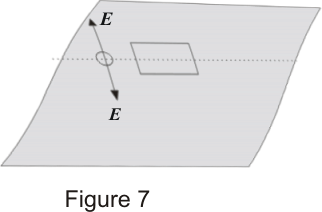• By symmetry we find that Eon either side of sheet must be perpendicular to the plane of the sheet, having same magnitude at all points equidistant from the sheet.
• No field lines crosses the side walls of the Gaussian pillbox i.e., component of E normal to walls of pillbox is zero.
• We now apply Gauss's law to this surfacein this case charge enclosed is
q=σA
where A is the area of end face of Gaussian pillbox.
• E points in the direction away from the plane i.e., E points upwards for points above the plane and downwards for points below the plane. Thus for top and bottom surfaces,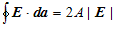thus
2A|E|=σA/ε0
or,
|E|=σ/2ε0
Here one important thing to note is that magnitude of electric field at any point is independent of the sheet and does not decrease inversely with the square of the distance. Thus electric field due to an infinite plane sheet of charge does not falls of at all.